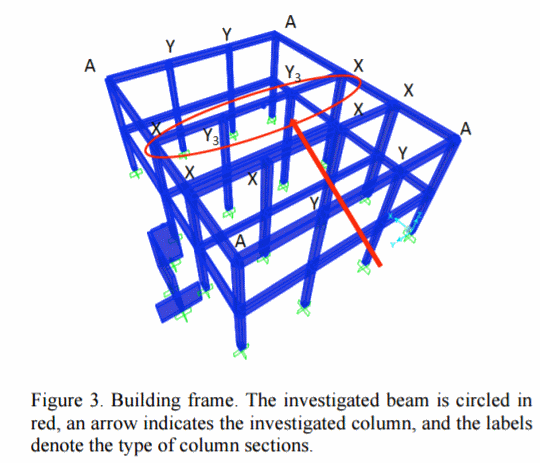# Combining Robustness and Reliability with Polynomial Chaos Techniques in Multiobjective Optimization Problems - Use of Percentiles

Author(s):
M. Marchi, L. Rizzian, E. Rigoni, R. Russo, A. Clarich (ESTECO)

CHALLENGE - Optimization results found with standard deterministic optimization techniques might not be the best ones in terms of robustness and reliability. A new method aims at estimating percentiles of functions of stochastic input variables by means of polynomial chaos expansion (PCE) techniques. The objective of this application is the seismic design of a building in the Italian town of Forni di Sopra (UD).

SOLUTION - In order to simulate the stresses in our two-storey building structure for given vertical loads and seismic actions, the software SAP2000 has been used. We have performed an optimization with two targets: the minimization of the overall weight of the most loaded coverage beam and the most stressed column and the maximization of the column constraint satisfaction w.r.t. compressive and flexural strengths due to the vertical loads. A deterministic optimization problem with 12 input variables and 3 input constant parameters has been considered. The problem has been optimized with NSGA-II. The results of the deterministic optimization have been used as starting points for the stochastic optimization run, again using NSGA-II algorithm with the same parameters as in the deterministic run. Optimization software modeFRONTIER is integrated with SAP2000 by means of an Excel file, which controls the SAP2000 model by using a Visual Basic macro.BENEFITS -  This method is effective in the solution of multiobjective optimization problems and is applicable to industrial engineering systems. It allows optimizing both the reliability and robustness of a system, since percentiles of constraint functions and expectation values or variances of performances can be optimized at the same time. Results show that the spread in the weight values obtained in the stochastic run is not big. In the deterministic run it is even smaller. Thus the two objectives considered in this calculations are correlated. Second, the average weight obtained in the stochastic optimization is greater than the weight obtained in the deterministic run meaning we get more reliable solutions at the expense of the weight.